Publicité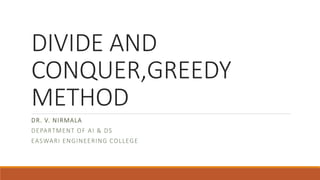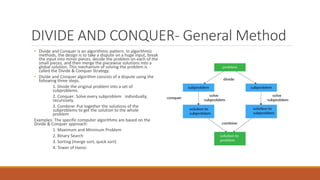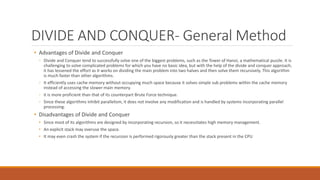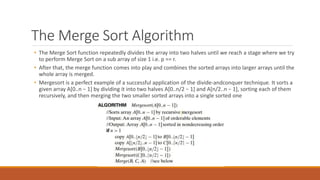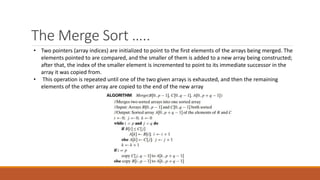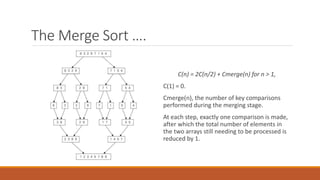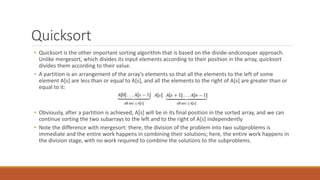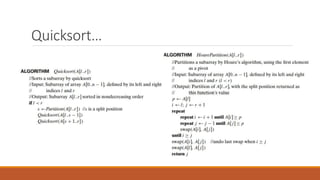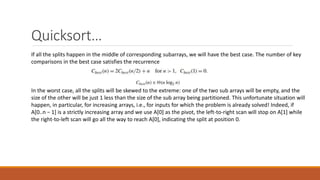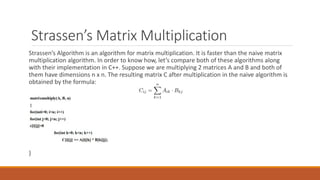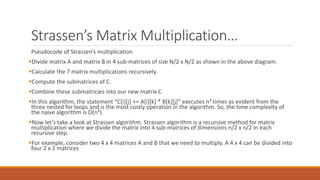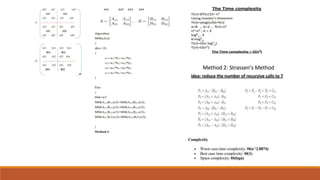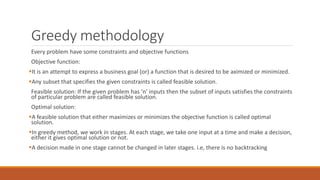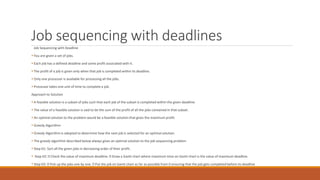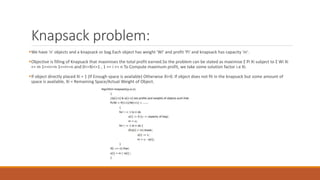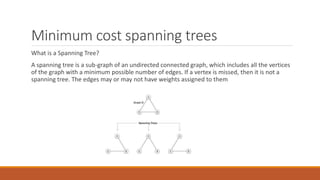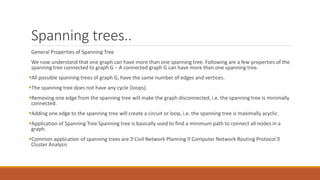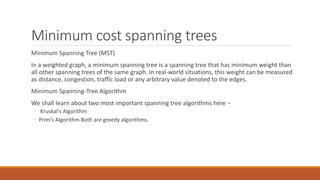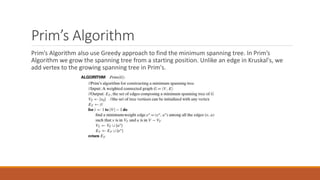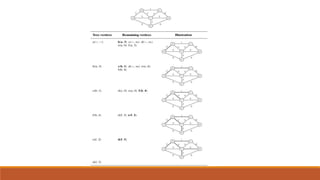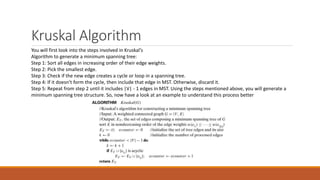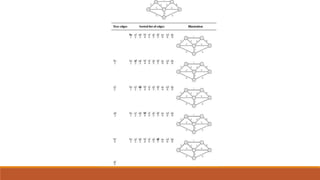1 sur 22
Publicité

### Divide and Conquer / Greedy Techniques

1. DIVIDE AND CONQUER,GREEDY METHOD DR. V. NIRMALA DEPARTMENT OF AI & DS EASWARI ENGINEERING COLLEGE
2. DIVIDE AND CONQUER- General Method • Divide and Conquer is an algorithmic pattern. In algorithmic methods, the design is to take a dispute on a huge input, break the input into minor pieces, decide the problem on each of the small pieces, and then merge the piecewise solutions into a global solution. This mechanism of solving the problem is called the Divide & Conquer Strategy. • Divide and Conquer algorithm consists of a dispute using the following three steps. 1. Divide the original problem into a set of subproblems. 2. Conquer: Solve every subproblem individually, recursively. 3. Combine: Put together the solutions of the subproblems to get the solution to the whole problem Examples: The specific computer algorithms are based on the Divide & Conquer approach: 1. Maximum and Minimum Problem 2. Binary Search 3. Sorting (merge sort, quick sort) 4. Tower of Hanoi.
3. DIVIDE AND CONQUER- General Method • Advantages of Divide and Conquer ◦ Divide and Conquer tend to successfully solve one of the biggest problems, such as the Tower of Hanoi, a mathematical puzzle. It is challenging to solve complicated problems for which you have no basic idea, but with the help of the divide and conquer approach, it has lessened the effort as it works on dividing the main problem into two halves and then solve them recursively. This algorithm is much faster than other algorithms. ◦ It efficiently uses cache memory without occupying much space because it solves simple sub problems within the cache memory instead of accessing the slower main memory. ◦ it is more proficient than that of its counterpart Brute Force technique. ◦ Since these algorithms inhibit parallelism, it does not involve any modification and is handled by systems incorporating parallel processing. • Disadvantages of Divide and Conquer • Since most of its algorithms are designed by incorporating recursion, so it necessitates high memory management. • An explicit stack may overuse the space. • It may even crash the system if the recursion is performed rigorously greater than the stack present in the CPU
4. The Merge Sort Algorithm • The Merge Sort function repeatedly divides the array into two halves until we reach a stage where we try to perform Merge Sort on a sub array of size 1 i.e. p == r. • After that, the merge function comes into play and combines the sorted arrays into larger arrays until the whole array is merged. • Mergesort is a perfect example of a successful application of the divide-andconquer technique. It sorts a given array A[0..n − 1] by dividing it into two halves A[0..n/2 − 1] and A[n/2..n − 1], sorting each of them recursively, and then merging the two smaller sorted arrays into a single sorted one
5. The Merge Sort ….. • Two pointers (array indices) are initialized to point to the first elements of the arrays being merged. The elements pointed to are compared, and the smaller of them is added to a new array being constructed; after that, the index of the smaller element is incremented to point to its immediate successor in the array it was copied from. • This operation is repeated until one of the two given arrays is exhausted, and then the remaining elements of the other array are copied to the end of the new array
6. The Merge Sort …. C(n) = 2C(n/2) + Cmerge(n) for n > 1, C(1) = 0. Cmerge(n), the number of key comparisons performed during the merging stage. At each step, exactly one comparison is made, after which the total number of elements in the two arrays still needing to be processed is reduced by 1.
7. Quicksort • Quicksort is the other important sorting algorithm that is based on the divide-andconquer approach. Unlike mergesort, which divides its input elements according to their position in the array, quicksort divides them according to their value. • A partition is an arrangement of the array’s elements so that all the elements to the left of some element A[s] are less than or equal to A[s], and all the elements to the right of A[s] are greater than or equal to it: • Obviously, after a partition is achieved, A[s] will be in its final position in the sorted array, and we can continue sorting the two subarrays to the left and to the right of A[s] independently • Note the difference with mergesort: there, the division of the problem into two subproblems is immediate and the entire work happens in combining their solutions; here, the entire work happens in the division stage, with no work required to combine the solutions to the subproblems.
8. Quicksort…
9. Quicksort… If all the splits happen in the middle of corresponding subarrays, we will have the best case. The number of key comparisons in the best case satisfies the recurrence In the worst case, all the splits will be skewed to the extreme: one of the two sub arrays will be empty, and the size of the other will be just 1 less than the size of the sub array being partitioned. This unfortunate situation will happen, in particular, for increasing arrays, i.e., for inputs for which the problem is already solved! Indeed, if A[0..n − 1] is a strictly increasing array and we use A as the pivot, the left-to-right scan will stop on A while the right-to-left scan will go all the way to reach A, indicating the split at position 0.
10. Strassen’s Matrix Multiplication Strassen’s Algorithm is an algorithm for matrix multiplication. It is faster than the naive matrix multiplication algorithm. In order to know how, let’s compare both of these algorithms along with their implementation in C++. Suppose we are multiplying 2 matrices A and B and both of them have dimensions n x n. The resulting matrix C after multiplication in the naive algorithm is obtained by the formula: }
11. Strassen’s Matrix Multiplication… Pseudocode of Strassen’s multiplication Divide matrix A and matrix B in 4 sub-matrices of size N/2 x N/2 as shown in the above diagram. Calculate the 7 matrix multiplications recursively. Compute the submatrices of C. Combine these submatricies into our new matrix C In this algorithm, the statement “C[i][j] += A[i][k] * B[k][j]” executes n³ times as evident from the three nested for loops and is the most costly operation in the algorithm. So, the time complexity of the naive algorithm is O(n³). Now let’s take a look at Strassen algorithm. Strassen algorithm is a recursive method for matrix multiplication where we divide the matrix into 4 sub-matrices of dimensions n/2 x n/2 in each recursive step. For example, consider two 4 x 4 matrices A and B that we need to multiply. A 4 x 4 can be divided into four 2 x 2 matrices
12. Greedy methodology Every problem have some constraints and objective functions Objective function: It is an attempt to express a business goal (or) a function that is desired to be aximized or minimized. Any subset that specifies the given constraints is called feasible solution. Feasible solution: If the given problem has ‘n’ inputs then the subset of inputs satisfies the constraints of particular problem are called feasible solution. Optimal solution: A feasible solution that either maximizes or minimizes the objective function is called optimal solution. In greedy method, we work in stages. At each stage, we take one input at a time and make a decision, either it gives optimal solution or not. A decision made in one stage cannot be changed in later stages. i.e, there is no backtracking
13. Job sequencing with deadlines Job Sequencing with Deadline  You are given a set of jobs.  Each job has a defined deadline and some profit associated with it.  The profit of a job is given only when that job is completed within its deadline.  Only one processor is available for processing all the jobs.  Processor takes one unit of time to complete a job. Approach to Solution  A feasible solution is a subset of jobs such that each job of the subset is completed within the given deadline.  The value of a feasible solution is said to be the sum of the profit of all the jobs contained in that subset.  An optimal solution to the problem would be a feasible solution that gives the maximum profit.  Greedy Algorithm-  Greedy Algorithm is adopted to determine how the next job is selected for an optimal solution.  The greedy algorithm described below always gives an optimal solution to the job sequencing problem  Step-01: Sort all the given jobs in decreasing order of their profit.  Step-02: Check the value of maximum deadline. Draw a Gantt chart where maximum time on Gantt chart is the value of maximum deadline.  Step-03: Pick up the jobs one by one. Put the job on Gantt chart as far as possible from 0 ensuring that the job gets completed before its deadline
14. Knapsack problem: We have 'n' objects and a knapsack or bag.Each object has weight 'Wi' and profit 'Pi' and knapsack has capacity 'm'. Objective is filling of Knapsack that maximises the total profit earned.So the problem can be stated as maximise Σ Pi Xi subject to Σ Wi Xi <= m 1<=i<=n 1<=i<=n and 0<=Xi<=1 , 1 <= i <= n To Compute maximum profit, we take some solution factor i.e Xi. If object directly placed Xi = 1 (If Enough space is available) Otherwise Xi=0. If object does not fit in the knapsack but some amount of space is available, Xi = Remaining Space/Actual Weight of Object.
15. Minimum cost spanning trees What is a Spanning Tree? A spanning tree is a sub-graph of an undirected connected graph, which includes all the vertices of the graph with a minimum possible number of edges. If a vertex is missed, then it is not a spanning tree. The edges may or may not have weights assigned to them
16. Spanning trees.. General Properties of Spanning Tree We now understand that one graph can have more than one spanning tree. Following are a few properties of the spanning tree connected to graph G − A connected graph G can have more than one spanning tree. All possible spanning trees of graph G, have the same number of edges and vertices. The spanning tree does not have any cycle (loops). Removing one edge from the spanning tree will make the graph disconnected, i.e. the spanning tree is minimally connected. Adding one edge to the spanning tree will create a circuit or loop, i.e. the spanning tree is maximally acyclic. Application of Spanning Tree Spanning tree is basically used to find a minimum path to connect all nodes in a graph. Common application of spanning trees are Civil Network Planning Computer Network Routing Protocol Cluster Analysis
17. Minimum cost spanning trees Minimum Spanning Tree (MST) In a weighted graph, a minimum spanning tree is a spanning tree that has minimum weight than all other spanning trees of the same graph. In real-world situations, this weight can be measured as distance, congestion, traffic load or any arbitrary value denoted to the edges. Minimum Spanning-Tree Algorithm We shall learn about two most important spanning tree algorithms here − ◦ Kruskal's Algorithm ◦ Prim's Algorithm Both are greedy algorithms.
18. Prim’s Algorithm Prim’s Algorithm also use Greedy approach to find the minimum spanning tree. In Prim’s Algorithm we grow the spanning tree from a starting position. Unlike an edge in Kruskal's, we add vertex to the growing spanning tree in Prim's.
19. Kruskal Algorithm You will first look into the steps involved in Kruskal’s Algorithm to generate a minimum spanning tree: Step 1: Sort all edges in increasing order of their edge weights. Step 2: Pick the smallest edge. Step 3: Check if the new edge creates a cycle or loop in a spanning tree. Step 4: If it doesn’t form the cycle, then include that edge in MST. Otherwise, discard it. Step 5: Repeat from step 2 until it includes |V| - 1 edges in MST. Using the steps mentioned above, you will generate a minimum spanning tree structure. So, now have a look at an example to understand this process better
Publicité